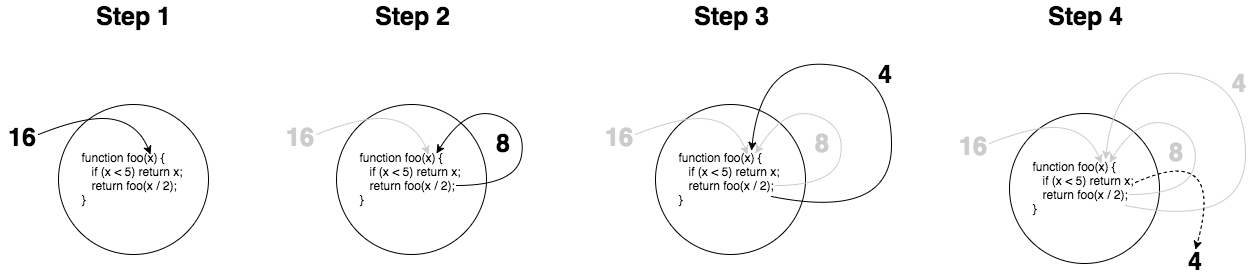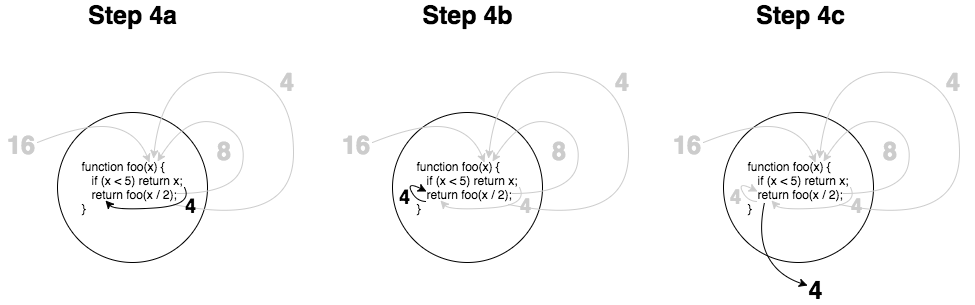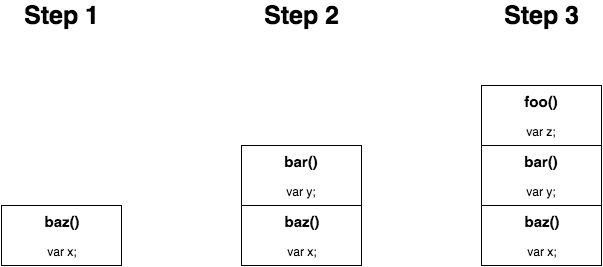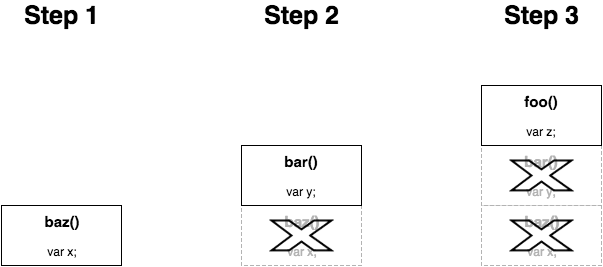# 第 9 章：递归

(本页剩余部分故意留白)

## 定义

``````function foo(x) {
if (x < 5) return x;
return foo( x / 2 );
}
````````````function isPrime(num,divisor = 2){
if (num < 2 || (num > 2 && num % divisor == 0)) {
return false;
}
if (divisor <= Math.sqrt( num )) {
return isPrime( num, divisor + 1 );
}

return true;
}
``````

``````fib( 0 ): 0
fib( 1 ): 1
fib( n ):
fib( n - 2 ) + fib( n - 1 )
``````

``````function fib(n) {
if (n <= 1) return n;
return fib( n - 2 ) + fib( n - 1 );
}
``````

### 相互递归

``````function isOdd(v) {
if (v === 0) return false;
return isEven( Math.abs( v ) - 1 );
}

function isEven(v) {
if (v === 0) return true;
return isOdd( Math.abs( v ) - 1 );
}
``````

``````function fib_(n) {
if (n == 1) return 1;
else return fib( n - 2 );
}

function fib(n) {
if (n == 0) return 0;
else return fib( n - 1 ) + fib_( n );
}
``````

### 为什么选择递归？

``````function sum(total,...nums) {
for (let i = 0; i < nums.length; i++) {
total = total + nums[i];
}

}

// vs

function sum(num1,...nums) {
if (nums.length == 0) return num1;
return num1 + sum( ...nums );
}
``````

## 声明式递归

``````function maxEven(...nums) {
var num = -Infinity;

for (let i = 0; i < nums.length; i++) {
if (nums[i] % 2 == 0 && nums[i] > num) {
num = nums[i];
}
}

if (num !== -Infinity) {
return num;
}
}
``````

``````maxEven( nums ):
maxEven( nums.0, maxEven( ...nums.1 ) )
``````

``````maxEven( 1, 10, 3, 2 ):
maxEven( 1, maxEven( 10, maxEven( 3, maxEven( 2 ) ) )
``````

``````function maxEven(num1,...restNums) {
var maxRest = restNums.length > 0 ?
maxEven( ...restNums ) :
undefined;

return (num1 % 2 != 0 || num1 < maxRest) ?
maxRest :
num1;
}
``````

``````maxEven( num1, ...restNums ):
maxEven( num1, maxEven( ...restNums ) )
``````

``````depth( node ):
1 + max( depth( node.left ), depth( node.right ) )
``````

``````function depth(node) {
if (node) {
let depthLeft = depth( node.left );
let depthRight = depth( node.right );
return 1 + max( depthLeft, depthRight );
}

return 0;
}
``````

## 栈、堆

``````function isOdd(v) {
if (v === 0) return false;
return isEven( Math.abs( v ) - 1 );
}

function isEven(v) {
if (v === 0) return true;
return isOdd( Math.abs( v ) - 1 );
}
``````

``````isOdd( 33333 );            // RangeError: Maximum call stack size exceeded
``````

``````function foo() {
var z = "foo!";
}

function bar() {
var y = "bar!";
foo();
}

function baz() {
var x = "baz!";
bar();
}

baz();
``````### 尾调用### 正确的尾调用 (PTC)

``````return foo( .. );
``````

``````foo();
return;

// 或

var x = foo( .. );
return x;

// 或

return 1 + foo( .. );
``````

`foo(..)` 运行结束之后 `1+` 这部分才开始执行，所以此时的堆栈帧依然存在。

``````return x ? foo( .. ) : bar( .. );
``````

`x` 进行条件判断之后，或执行 `foo(..)`，或执行 `bar(..)`，不论执行哪个，返回结果都会被 `return` 返回掉。这个例子符合 PTC 规范。

## 重构递归

### 更换堆栈

``````function sum(num1,...nums) {
if (nums.length == 0) return num1;
return num1 + sum( ...nums );
}
``````

``````function sum(result,num1,...nums) {
// ..
}
``````

``````"use strict";

function sum(result,num1,...nums) {
result = result + num1;
if (nums.length == 0) return result;
return sum( result, ...nums );
}
``````

``````sum( /*initialResult=*/0, 3, 1, 17, 94, 8 );        // 123
``````

``````"use strict";

function sumRec(result,num1,...nums) {
result = result + num1;
if (nums.length == 0) return result;
return sumRec( result, ...nums );
}

function sum(...nums) {
return sumRec( /*initialResult=*/0, ...nums );
}

sum( 3, 1, 17, 94, 8 );                                // 123
``````

``````"use strict";

function sum(...nums) {
return sumRec( /*initialResult=*/0, ...nums );

function sumRec(result,num1,...nums) {
result = result + num1;
if (nums.length == 0) return result;
return sumRec( result, ...nums );
}
}

sum( 3, 1, 17, 94, 8 );                                // 123
``````

``````"use strict";

var sum = (function IIFE(){

return function sum(...nums) {
return sumRec( /*initialResult=*/0, ...nums );
}

function sumRec(result,num1,...nums) {
result = result + num1;
if (nums.length == 0) return result;
return sumRec( result, ...nums );
}

})();

sum( 3, 1, 17, 94, 8 );                                // 123
``````

``````"use strict";

function sum(result,num1,...nums) {
result = result + num1;
if (nums.length == 0) return result;
return sum( result, ...nums );
}

sum( /*initialResult=*/0, 3, 1, 17, 94, 8 );        // 123
``````

``````"use strict";

function sum(num1,num2,...nums) {
num1 = num1 + num2;
if (nums.length == 0) return num1;
return sum( num1, ...nums );
}

sum( 3, 1, 17, 94, 8 );                                // 123
``````

1. 首先对前两个参数 `num1``num2` 进行对比。
2. 如果 `num1` 是偶数，并且 `num1` 大于 `num2``num1` 保持不变。
3. 如果 `num2` 是偶数，把 `num2` 赋值给 `num1`
4. 否则的话，`num1` 等于 `undefined`
5. 如果除了这两个参数之外，还存在其它参数 `nums`，把它们与 `num1` 进行递归对比。
6. 最后，不管是什么值，只需返回 `num1`

``````"use strict";

function maxEven(num1,num2,...nums) {
num1 =
(num1 % 2 == 0 && !(maxEven( num2 ) > num1)) ?
num1 :
(num2 % 2 == 0 ? num2 : undefined);

return nums.length == 0 ?
num1 :
maxEven( num1, ...nums )
}
``````

### 后继传递格式 （CPS）

`fib(..)` 做如下修改：

``````"use strict";

function fib(n,cont = identity) {
if (n <= 1) return cont( n );
return fib(
n - 2,
n2 => fib(
n - 1,
n1 => cont( n2 + n1 )
)
);
}
``````

### 弹簧床

``````function trampoline(fn) {
return function trampolined(...args) {
var result = fn( ...args );

while (typeof result == "function") {
result = result();
}

return result;
};
}
``````

``````var sum = trampoline(
function sum(num1,num2,...nums) {
num1 = num1 + num2;
if (nums.length == 0) return num1;
return () => sum( num1, ...nums );
}
);

var xs = [];
for (let i=0; i<20000; i++) {
xs.push( i );
}

sum( ...xs );                    // 199990000
``````